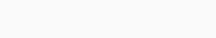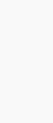# Making changes on a pivot table

## Making changes on a pivot table

Contents

Problem Description:

I have the following query:

``````
SELECT CASE GROUPING_ID(status, CASE WHEN status IN (2, 4, 5) THEN 1 ELSE 0 END)
WHEN 0
THEN TO_CHAR(status)
WHEN 2
THEN 'subtotal'
ELSE 'total'
END AS status,
COUNT(CASE user_type WHEN 1 THEN 1 END) AS "1",
COUNT(CASE user_type WHEN 2 THEN 1 END) AS "2",
COUNT(CASE user_type WHEN 3 THEN 1 END) AS "3",
COUNT(CASE user_type WHEN 5 THEN 1 END) AS "5",
COUNT(*) AS total
FROM   (Select STATUS,USER_TYPE
FROM TRANSACTIONS tr  join TRANSACTION_STATUS_CODES sc on sc.id = tr.user_type join
TRANSACTION_USER_TYPES ut on ut.id=tr.user_type
WHERE tr.status!=1 AND Tr.Update_Date BETWEEN     TO_DATE('2022-01-01 00:00:00', 'yyyy-mm-dd HH24:MI:SS')     AND TO_DATE('2022-11-13 23:59:59', 'yyyy-mm-dd HH24:MI:SS'))
GROUP BY CUBE(status,CASE WHEN status IN (2, 4, 5) THEN 1 ELSE 0 END)
HAVING GROUPING_ID(status, CASE WHEN status IN (2, 4, 5) THEN 1 ELSE 0 END) IN (0, 3)
OR     (   GROUPING_ID(status, CASE WHEN status IN (2, 4, 5) THEN 1 ELSE 0 END) = 2
AND CASE WHEN status IN (2, 4, 5) THEN 1 ELSE 0 END = 1 );
``````

which displays:

[with inner query giving ( thats 10 out of 36rows):anyways.. I’ve been asked to change the inner query to a better organized one, so I came up with the following pivot table ( which I want it to be my new inner query ):

``````SELECT p.*
FROM   (
SELECT user_type,
status
FROM   transactions
WHERE  status !=1
AND    Update_Date >= DATE '2022-01-01'
AND    Update_Date <  DATE '2022-11-14'
) PIVOT (
COUNT(*)
FOR user_type IN (1,2,3,5)
) p
ORDER BY status asc;
``````

which gives:what I need to try and achieve is the sum of subtotal/total of rows aswell as total of column( as I have in the first table ), and when I try to make it an inner query the outer query doesnt recognize anything..is that achievable without changing the entire query?
or else, what is the best approach to achieve that?

## Solution – 1

`CUBE` does what you want in a single query and was covered in my previous answer.

You cannot use `PIVOT` to do what you want in a single query; instead you would need to use multiple queries joined together with `UNION ALL` to calculate the status, sub-total and total parts (which is likely to be much less efficient):

``````SELECT TO_CHAR(status) AS status,
"1",
"2",
"3",
"5",
"1" + "2" + "3" + "5" AS total
FROM   table_name
PIVOT ( COUNT(*) FOR user_type IN (1,2,3,5) )
UNION ALL
SELECT 'SUB_TOTAL',
"1",
"2",
"3",
"5",
"1" + "2" + "3" + "5" AS total
FROM   (SELECT user_type FROM table_name WHERE status IN (2,4,5))
PIVOT ( COUNT(*) FOR user_type IN (1,2,3,5) )
UNION ALL
SELECT 'TOTAL',
"1",
"2",
"3",
"5",
"1" + "2" + "3" + "5" AS total
FROM   (SELECT user_type FROM table_name)
PIVOT ( COUNT(*) FOR user_type IN (1,2,3,5) )
``````

or:

``````SELECT TO_CHAR(status) AS status,
COUNT(CASE user_type WHEN 1 THEN 1 END) AS "1",
COUNT(CASE user_type WHEN 2 THEN 1 END) AS "2",
COUNT(CASE user_type WHEN 3 THEN 1 END) AS "3",
COUNT(CASE user_type WHEN 5 THEN 1 END) AS "5",
COUNT(*) AS total
FROM   table_name
GROUP BY status
UNION ALL
SELECT 'SUB_TOTAL',
COUNT(CASE user_type WHEN 1 THEN 1 END) AS "1",
COUNT(CASE user_type WHEN 2 THEN 1 END) AS "2",
COUNT(CASE user_type WHEN 3 THEN 1 END) AS "3",
COUNT(CASE user_type WHEN 5 THEN 1 END) AS "5",
COUNT(*) AS total
FROM   table_name
WHERE  status IN (2,4,5)
UNION ALL
SELECT 'TOTAL',
COUNT(CASE user_type WHEN 1 THEN 1 END) AS "1",
COUNT(CASE user_type WHEN 2 THEN 1 END) AS "2",
COUNT(CASE user_type WHEN 3 THEN 1 END) AS "3",
COUNT(CASE user_type WHEN 5 THEN 1 END) AS "5",
COUNT(*) AS total
FROM   table_name
``````

Which, for the sample data, (also from my previous answer):

``````CREATE TABLE table_name (status, user_type) AS
SELECT 2, 1 FROM DUAL CONNECT BY LEVEL <=  3 UNION ALL
SELECT 4, 1 FROM DUAL CONNECT BY LEVEL <= 13 UNION ALL
SELECT 5, 1 FROM DUAL CONNECT BY LEVEL <=  1 UNION ALL
SELECT 3, 1 FROM DUAL CONNECT BY LEVEL <=  5 UNION ALL
SELECT 3, 5 FROM DUAL CONNECT BY LEVEL <=  1 UNION ALL
SELECT 0, 1 FROM DUAL CONNECT BY LEVEL <=  4 UNION ALL
SELECT 0, 5 FROM DUAL CONNECT BY LEVEL <=  8;
``````

Both output the same as using a single query with `CUBE`:

STATUS1235TOTAL
230003
41300013
0400812
510001
350016
SUB_TOTAL1700017
TOTAL2600935

If you did want to do it without `CUBE` then you can generate extra rows before pivoting (however, I think `CUBE` will still be more efficient):

``````SELECT title AS status,
"1",
"2",
"3",
"5",
"1" + "2" + "3" + "5" AS total
FROM   (
SELECT l.*, t.user_type
FROM   table_name t
CROSS JOIN LATERAL (
SELECT t.status, TO_CHAR(t.status) AS title, 1 AS priority FROM DUAL UNION ALL
SELECT NULL,     'SUB_TOTAL',                2 FROM DUAL WHERE t.status IN (2,4,5) UNION ALL
SELECT NULL,     'TOTAL',                    3 FROM DUAL
) l
)
PIVOT ( COUNT(*) FOR user_type IN (1,2,3,5) )
ORDER BY priority, status
``````

Which produces the same output.

fiddle

Rate this post
We use cookies in order to give you the best possible experience on our website. By continuing to use this site, you agree to our use of cookies.